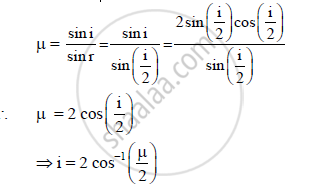HSC Science (Electronics) 12th Board ExamMaharashtra State Board
Share

# A Ray of Light Passes from a Vacuum to a Medium of Refractive Index (μ). the Angle of Incidence is Found to Be Twice the Angle of Refraction. the Angle of Incidence is - HSC Science (Electronics) 12th Board Exam - Physics

#### Question

A ray of light passes from a vacuum to a medium of refractive index (μ). The angle of
incidence is found to be twice the angle of refraction. The angle of incidence is _______.

A) cos^(-1)(mu/2)

B) cos−1(μ)

C)  2 cos^(-1) (mu/2)

D) 2 sin^(-1) (mu/2)

#### Solution

For i = 2rIs there an error in this question or solution?

#### Video TutorialsVIEW ALL 

Solution A Ray of Light Passes from a Vacuum to a Medium of Refractive Index (μ). the Angle of Incidence is Found to Be Twice the Angle of Refraction. the Angle of Incidence is Concept: Polarisation.
S